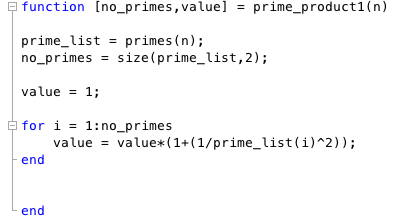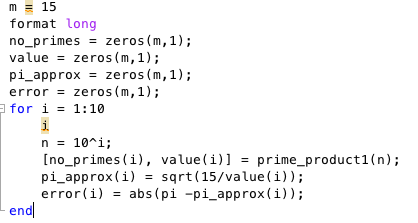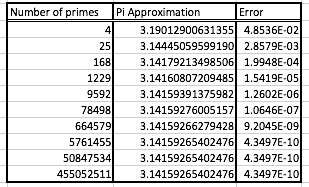Categories

# Pi Day 2016

Following on from last years Pi day post, I thought I would briefly post about another series method for calculating pi.

I came across this cool infinite product involving the primes a few years ago, and was recently reminded of it when I saw Matt Parker (@standupmaths) in Nottingham recently.

This infinite product is due to the genius Ramanujan and was published in the Journal of the Indian Mathematical Society in 1913.The product I am interested in is numbered (5) in the article above, namely $$\left(1+\frac{1}{2^2} \right) \left(1+\frac{1}{3^2} \right)\left(1+\frac{1}{5^2} \right)\left(1+\frac{1}{7^2} \right) \cdots = \frac{15}{\pi^2}$$. If $$P_n$$ denotes the partial product up to the $$n$$th prime then we can say that $$P_n \approx \frac{15}{\pi^2}$$ and so $$\pi \approx \sqrt{\frac{15}{P_n}}$$.

I was interested in how quickly this converged so I coded it up in MATLAB (in an easy to understand style, not a way to maximise speed)In the above bit of code the MATLAB function $$\texttt{primes}$$ produces a list of all prime numbers below the integer n. Thus, the code doesn’t directly compute the product up to the $$n$$th prime in the sense that you might expect. I then called this function from a script file to generate a list of outputs.With this we obtain the following table of results:Broadly speaking we see that for an increase in an order of magnitude of the number of primes we get an extra correct decimal digit of pi (it’s too late on a Sunday when I am writing this for me to do a proper convergence analysis). I believe the issues in the last three lines are likely due to the limitations of using floating point arithmetic, but I have not had a chance to try this method out using a variable precision arithmetic library.

If you have MATLAB and want to try it out for your self the files are available from my website at prime_pi.m and prime_product1.m.